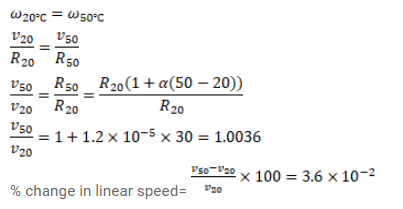# A circular disc made of iron is rotated about its axis`
Question:

A circular disc made of iron is rotated about its axis at a constant velocity $\omega$. Calculate the percentage change in the linear speed of the particle of the rim as the disc is slowly heated from $20^{\circ} \mathrm{C}$ to $50^{\circ} \mathrm{C}$ keeping the angular velocity constant. Coefficient of linear expansion of iron= $1.2 \times 10^{-5} /{ }^{\circ} \mathrm{C}$.

Solution: